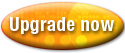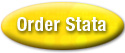Home  /  Products  /  Stata 9  /  Multivariate methods

#### This page contains only historical information and is not about the current release of Stata. Please see our Stata 18 page for information on the current version of Stata.## Multivariate methods

Stata 9 includes four new methods for analyzing multivariate data, and it includes many extensions to existing methods, especially for factor and principal-component analysis.

Stata now performs multidimensional scaling (MDS) on raw data, on proximity matrices, and on proximity datasets; 33 similarity/dissimilarity measures are supported. Configuration graphs and Shepard diagrams are also available.

Stata now performs two-way correspondence analysis on datasets or on count matrices. You can obtain row and column profiles, chi-squared distances, and inertias. Biplots and dimensional-projection plots are also available.

Stata now performs Procrustean transformations for comparing the similarity between two sets of variables or datasets. Overlay plots are available. Stata now performs biplot analysis and produces two-dimensional biplots of results. Variables are plotted as arrows—the cosine of the angle between the arrows approximates the correlation—and observations are plotted so that distances are approximately preserved.

Stata’s factor analysis and principal-component analysis commands now analyze correlation matrices, as well as raw data, and provide over 20 oblique and orthogonal rotations.

Stata’s PCA command now will compute the VCE of the eigenvalues and eigenvectors, assuming multivariate normality, giving you access to most of Stata’s postestimation facilities—including tests—and giving you CIs on scree plots.

Here are all the details.

### New methods

In addition to reading about the new methods, be sure to check the postestimation documentation for the multivariate estimators you use to learn about many important new features. In particular, all the multivariate commands make extensive use of new command estat for providing additional statistics and results after estimation.

• New commands mds, mdslong, and mdsmat perform classic metric multidimensional scaling: mds performs the scaling with respect to the distances (dissimilarities) between observations, mdslong performs the scaling on a long dataset where each observation represents the distance between two points or objects, and mdsmat performs the scaling on a matrix of distances. See [MV] mds, [MV] mdslong, and [MV] mdsmat.

mds supports all 33 similarity/dissimilarity measures available in Stata; see [MV] measure_option.

The following new estat commands work after mds, mdslong, and mdsmat and provide additional statistics and results:

• estat config also reports the coordinates of the approximating configuration.

• estat correlations reports the Pearson and Spearman correlations between the dissimilarities and the approximating distances for each object.

• estat pairwise reports a set of statistics for each pairwise comparison; it reports the dissimilarities, the approximating distances, and the raw residuals.

• estat quantiles reports the quantiles of the residuals for each observation (after mds) or object (after mdslong or mdsmat).

• estat stress reports the Kruskal stress (loss) measure between the transformed dissimilarities and fitted distances for each object.

In addition, there are two new commands for graphing results from a multidimensional scaling:

• mdsconfig plots the approximating Euclidean configuration of the first two dimensions; see [MV] mds postestimation.

• mdsshepard produces a Shepard diagram of the dissimilarities against the approximating Euclidean distances; see [MV] mds postestimation.

predict after any multidimensional-scaling command produces

• variables containing the approximating configuration (predict newvarlist , config);

• variables containing the dissimilarity, distance, and raw residuals (predict newvarlist , pairwise)

• New commands ca and camat perform two-way correspondence analysis using any of several available forms of normalization. ca performs the analysis on the cross-tabulation of two categorical variables; camat performs the analysis on a matrix of counts; see [MV] ca for more information on both commands.

The following new estat commands work after ca and camat and provide additional statistics and results

• estat coordinates reports the coordinates in both the row space and the column space.

• estat distances reports the chi-squared distances between the row profiles and between the column profiles, including the distances to the marginal distributions (commonly called centers). Both observed and fitted profiles are available.

• estat inertia reports the inertia contributions of the individual cells.

• estat profiles reports the row profiles and column profiles—the conditional distributions, given the other dimension.

• estat summarize reports summary information of the row and column variables over the estimation sample.

• estat table reports the fitted correspondence table, the observed "correspondence" table, or the expected table under the assumption of independence.

In addition, there are two new commands for graphing results from a correspondence analysis:

• cabiplot produces a biplot of each row category and each column category; see [MV] ca postestimation.

• caprojection produces a graph that shows the ordering of row categories and column categories on each principal dimension of the analysis. Each principal dimension is represented by a vertical line; markers are plotted on the lines where the row categories and column categories project onto the dimensions; see [MV] ca postestimation.

predict after ca and camat computes fitted values and row or column scores for any dimension; see [MV] ca postestimation.

• The new command procrustes performs Procrustean analysis for comparing and measuring the similarity between two sets of variables: source and target. Two datasets can also be compared if the datasets are first merged by record.

The following new estat commands work after procrustes and provide additional statistics and results:

• estat compare reports fit statistics of the three transformations available in Procrustean analysis: orthogonal, oblique, and unrestricted.

• estat mvreg reports the multivariate regression that is related to the current Procrustean analysis.

• estat summarize reports summary information of the two sets of variables over the estimation sample.

New command procoverlay after procrustes creates an overlay graph comparing the target variables with the fitted values derived from the source variables; see [MV] procrustes postestimation.

predict after procrustes produces fitted values for all variables, residuals for all variables, or residual sums of squares for a specified target variable; see [MV] procrustes postestimation.

• New command biplot performs a biplot analysis of a dataset and produces a two-dimensional biplot of the results. A biplot simultaneously displays the observations (rows) and the relative positions of the variables (columns). Observations are projected to two dimensions such that the distance between the observations is approximately preserved. The variables are plotted as arrows, with the cosine of the angle between the arrows approximating the correlation between the variables. See [MV] biplot.

• New command tetrachoric computes a tetrachoric correlation matrix for a set of binary variables. tetrachoric is documented in [R] but often used in multivariate analyses; see [R] tetrachoric.

tetrachoric results can be used in subsequent factor analyses or principal component analyses using the new [MV] factormat and [MV] pcamat commands.

• Existing command canon now allows analysis and presentation of more than one linear combination and has new options for reporting the raw or standardized coefficients and for reporting significance tests of the canonical correlations; see [MV] canon.

The following new estat commands work after canon and provide additional statistics and results:

• estat correlations reports the correlations among all variables.

• Existing command cluster dendrogram has many new features, including horizontal dendrograms and the ability to label branch counts. The look of the graph can now be changed (titles, axes, colors, etc.); see [MV] cluster dendrogram.

• The existing hierarchical cluster commands have new option measure() that specifies the proximity measure to use in computing dissimilarities between observations. Any of 33 measures may be specified; see [MV] measure_option. Previously most of the measures were available under other option names; those options continue to work but are undocumented. See [MV] cluster.

• Existing command cluster stop has new option varlist() that specifies alternative variables to use when computing the stopping rules; see [MV] cluster stop.

### Analysis of proximity matrices

All of Stata’s multivariate analysis facilities that rely on pairwise comparisons of distance, similarity, dissimilarity, covariance, correlation, or other proximity measures can now work directly with proximity matrices that you compute or obtain from other sources.

Previously, all these facilities worked only with raw datasets. The new commands implement analyses on matrices. They share the common ability to accept either full matrices or vectors representing the lower or upper triangle of a symmetric proximity matrix.
• New command clustermat extends all of Stata’s hierarchical clustering facilities to the analysis of matrices of a dissimilarity measure (sometimes called a distance or proximity measure). This includes all seven linkage methods and the ability to create dendrograms of the results; see [MV] clustermat.

• New command factormat performs factor analysis on a matrix of correlations, extending all the new and previously available capabilities of the existing command [MV] factor to precomputed matrices of correlations; see [MV] factormat.

• New command pcamat performs principal component analysis on an existing correlation or covariance matrix; see [MV] pcamat.

• New matrix subcommand dissimilarity computes similarity, dissimilarity, or distance matrices using any of 19 proximity measures for continuous data and 14 measures for binary data; see [MV] measure_option and see [MV] matrix dissimilarity.

### Additions to factor and principal component analysis

In addition to allowing direct analysis of correlation and covariance matrices using factormat and pcamat, Stata’s factor analysis and principal components analysis (PCA) methods have been expanded, particularly through the addition of postestimation commands for reporting and graphing results.
• Command factor has new reporting option altdivisor, that specifies the trace of the correlation matrix be used as the divisor for proportions, rather than the default (the sum of all eigenvalues).

• New estat commands for use after factor and factormat provide additional statistics and results:

• estat common reports the correlation matrix of the common factors and is more of interest after oblique rotations.

• estat factors reports model-selection criteria (AIC and BIC) over all the factors retained in an analysis.

• estat structure reports the factor structure—the correlations between the variables and the common factors.

• Existing command pca allows several new options:

• Option vce(normal) computes the VCE of the eigenvalues and eigenvectors, assuming multivariate normality.

This gives you access to many of Stata’s postestimation facilities for analyzing estimation results, including tests of eigenvalue and eigenvector significance, tests of linear and nonlinear combinations ([R] test and [R] testnl), linear and nonlinear combinations with confidence intervals ([R] lincom and [R] nlcom), and nonlinear predictions with confidence intervals ([R] predictnl).

vce(normal) also produces the ingredients for adding confidence intervals to screeplots; see [MV] screeplot.

• Options level(), blanks(), novce, and norotated allow more flexible control of the displayed results.

• Option components(#) specifies the number of components to retain and is a synonym for old option factor().

• Options tol() and ignore provide advanced control for computationally difficult problems.

• New estat commands for use after pca and pcamat provide additional statistics and results:

• estat rotatecompare reports the unrotated (principal) components next to the most recent rotated components.

• New estat commands for use after any factor analysis or any principal components analysis (that is, after factor or factormat or after pca or pcamat) provide additional statistics and results:

• estat anti reports the anti-image correlation and anti-image covariance matrices.

• estat kmo reports the Kaiser–Meyer–Olkin measure of sampling adequacy.

• estat residuals reports the difference between the observed correlation or covariance matrix and the fitted (reproduced) matrix using the retained factors.

• estat smc reports the squared multiple correlations (SMC) between each variable and all other variables. SMC is a theoretical lower bound for communality, so it is an upper bound for the unexplained variance.